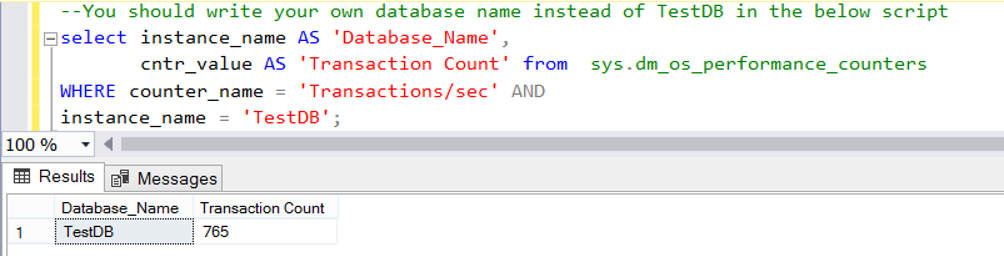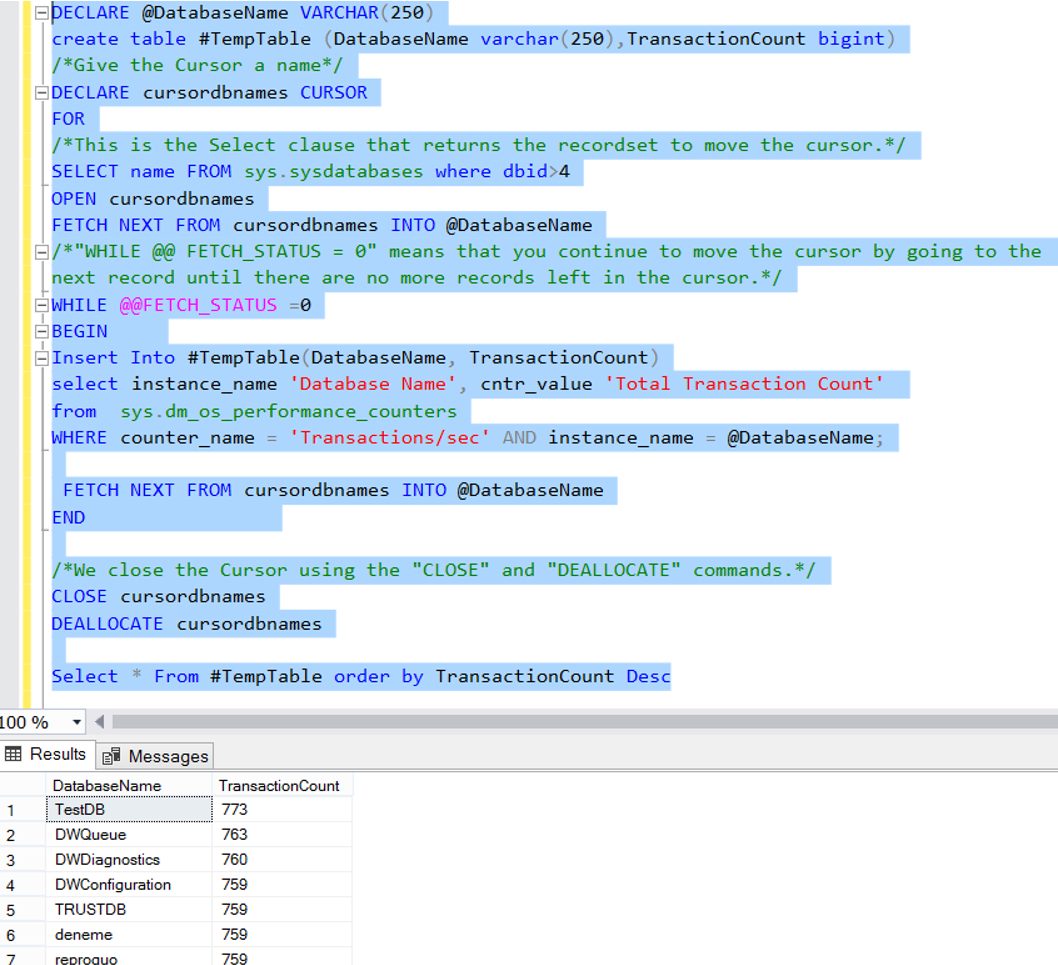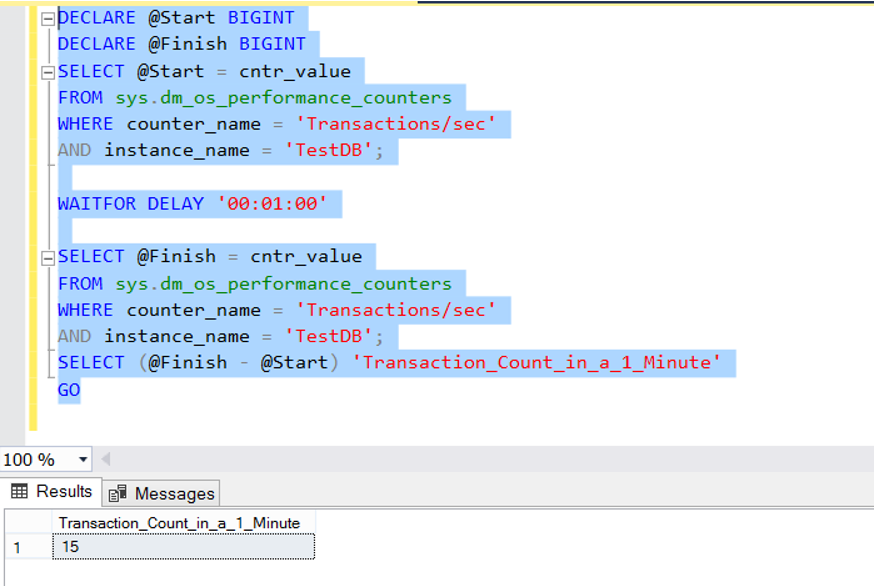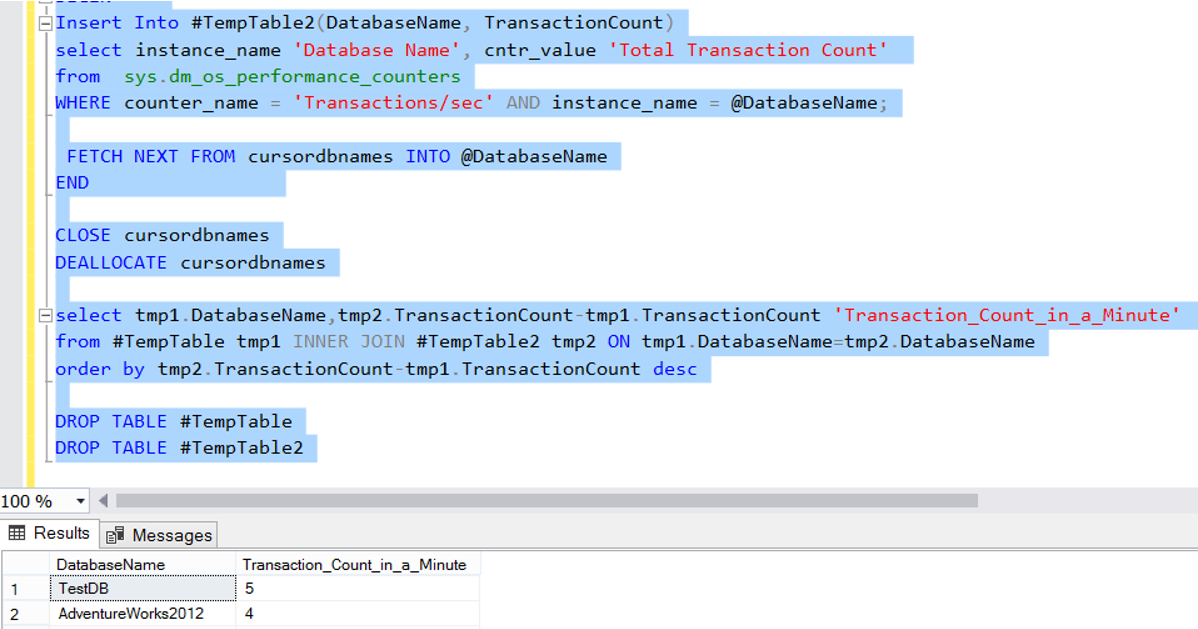Thursday , September 28 2023

# How To Calcutale Database Transaction Count in SQL Server

Transaction count shows that how heavily the database is used. Some third-party applications can show the transaction count graphs of the databases. But each company may not buy these applications. This article will be useful if you would like to find out manually the transaction count in your system.

You can find the database transaction count since the start of SQL Server by using the following script.You can find all database’s transaction count in the instance since the start of SQL Server by using the following script.You can also calculate the database transaction count in a specific time interval. With the following script, let’s calculate a database transaction count in a specific time interval. In Script, we set this time interval as 1 minute. You can extend this interval if you like.You can use the following script to calculate the transaction count in a specific time interval(Its 1 minute in our example) for all databases on Instance.